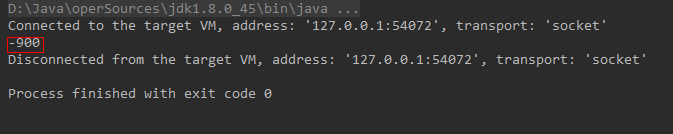• 2021-03-20 16:07:17

Java 如何求数组内的最小值
这里的知识点包括：

1. List 没有直接对应的 min，需要先转换为 ArrayList。
2. Collections.min() 可以获取最小值。
3. List 转换为 ArrayList，需要先 new 一个新的 ArrayList。
4. Arrays.asList() 可以配合 ArrayList() 方法，将 List 转换为 ArrayList。
ArrayList<Integer> arrayListB = new ArrayList<>(Arrays.asList(listA));
int minValue = Collections.min(arrayListB);编程语言
更多相关内容
• 主要介绍了JAVA得到数组中最大值和最小值的简单实例,需要的朋友可以参考下
• 出所有元素的最大最小值总和均值 public class MaxMinSumMean { public static void main(String[] args){ int[] arr = new int; for(int i = 0; i < arr.length; i++){ arr[i] = (int)(Math...

定义一个int型的一维数组，包含10个元素，分别赋一些随机数（两位数字）
求出所有元素的最大值最小值总和均值

public class MaxMinSumMean {
public static void main(String[] args){
int[] arr = new int;

for(int i = 0; i < arr.length; i++){
arr[i] = (int)(Math.random() * (99 - 10 + 1) + 10 );
// 生成两位数即[10,99]
// 利用公式[a,b]之间: (int)(Math.random() * (b - a + 1) + a )
}

// 遍历并输出数组
for(int i = 0; i < arr.length; i++){
System.out.print(arr[i] + "\t");
}
System.out.println();

// MAX
int maxValue = arr; // 不要设成0或其他数字，无法保证其足够小
for(int i = 0; i < arr.length; i++){
if(maxValue < arr[i]){
maxValue = arr[i];
}
}
System.out.println("最大值：" + maxValue);

// MIN
int minValue = arr; // 不要设成0或其他数字，无法保证其足够小
for(int i = 0; i < arr.length; i++){
if(minValue > arr[i]){
minValue = arr[i];
}
}
System.out.println("最小值：" + minValue);

// SUM
int sumValue = 0;
for(int i = 0; i < arr.length; i++){
sumValue += arr[i];
}
System.out.println("总和：" + sumValue);

// MEAN
int meanValue = sumValue/arr.length;
System.out.println("均值：" + meanValue);
}
}


展开全文算法
• 主要介绍了java 求解二维数组最小值的相关资料,需要的朋友可以参考下
• 1.实现代码 /** * 需求: 获取数组中最小值 */ public class ArrayUtil { public static void getMin(int[] arr) { // 假设第一位是最小值 int min = arr; for(int index = 0; index ; index ++) { // 判断数组...

### 1.实现代码

/**
* 需求: 获取数组中的最小值
*/
public class ArrayUtil {

public static void getMin(int[] arr) {
// 假设第一位是最小值
int min = arr;
for(int index = 0; index < arr.length; index ++) {
// 判断数组元素的最小值
if(arr[index] < min) {
// 把最小值存储Min变量
min = arr[index];
}
}
System.out.println(min); //-900
}
public static void main(String[] args) {
// 定义一个实际数组
int[] arr = new int[]{30,70,-900,9,60};
// 把数组传给getMin方法
getMin(arr);
}
}

### 2.输出结果展开全文算法 排序算法
• ## java求数组中最大值最小值

万次阅读 多人点赞 2017-07-18 22:22:11
算法描述 默认最大值是第一个元素 默认最小值也是第一个元素 遍历数组元素,比较数组元素的值和默认的最大值,... 另一种方式是记下最大最小值数组中的下标，再通过下标输出最大最小值，推荐使用这种方式. 代码...

## 算法描述

默认最大值是第一个元素

默认最小值也是第一个元素

遍历数组元素,比较数组元素的值和默认的最大值,如果数组元素的值比默认最大值还要大,则用数组元素的值作为默认的最大值,这样遍历一遍之后就可以求出最大值了.求最小值也是同样.

这里有两种方式来求记录最大最小值:

• 一种是记下最大值和最小值
• 另一种方式是记下最大值最小值在数组中的下标，再通过下标输出最大最小值，推荐使用这种方式.

# 代码

具体看下面的代码

public class MaxAndMin {
public static void main(String[] args) {
int[] array={1,3,67,34,23,89,56,34,21};//定义静态数组
System.out.println("----------方法1：-----------");
int max=array;//默认第一个最大
int min=array;//默认第一个最小
for(int i=1;i<array.length;i++)
{
if(array[i]>max)
{
max=array[i];//如果有比max大的数就让max记录下大的数
}
if(array[i]<min)
{
min=array[i];//如果有比min小的数就让min记录下小的数
}

}
System.out.println("max="+max);
System.out.println("min="+min);
System.out.println("---------------方法2：--------------");
int maxlocation=0;//默认第一个最大
int minlocation=0;//默认第一个
for(int i=0;i<array.length;i++)
{
if(array[i]>array[maxlocation])
{
maxlocation=i;//如果有比max大的数就让max记录下大的数
}
if(array[i]<array[minlocation])
{
minlocation=i;//如果有比min小的数就让min记录下小的数
}
}
System.out.println("max="+array[maxlocation]+" 位置："+maxlocation);
System.out.println("max="+array[minlocation]+" 位置："+minlocation);
}
}

展开全文• DK8特性：java最大值（整数数组）： int[] A = {6,7,8,2}; int maxVal = Arrays.stream(A).max().getAsInt(); 1 2 JDK8特性：java最小值（整数数组）： int[] A = {6,4,5}; int minVal = Arrays.stream(A)....
• 默认最大值是第一个元素默认最小值也是第一个元素遍历数组元素,比较数组元素的值和默认的最大值,如果数组元素的值比默认最大值还要大,则用数组元素的值作为默认的最大值,这样遍历一遍之后就可以 public class ...算法
• 展开全部java中随便定义一个数组时，需要先排序之后，然后输出最大最小值，可以使用sort类进行排序，实例如下32313133353236313431303231363533e78988e69d8331333337616530：publicclassArrDemo{...
• 声明一个整型数组，包含10个元素，给数组中的每个元素赋0~99的随机整数，数组中最大值、最小值 具体代码如下： package day05; import java.util.Arrays; public class MaxOfArray { public static void ...
• "\ndouble[]类型的数组元素的最大值为：" +rst[ 0 ]); System. out .println( "double[]类型的数组元素的最小值为：" +rst[ 1 ]); System. out .println( "double[]类型的数组元素的平均值为：" +rst[ 2 ]); } ...
• 主要介绍了java 输入一个数字组成的数组，输出该数组最大值和最小值,需要的朋友可以参考下
• C语言程序设计-把20个随机数存入一个数组，然后输出该数组中最小值;其中确定最小值的下标的操作在fun函数实现，请给出该函数的定义;.c
• java利用数组求平均值,最大值,最小值。需要的朋友可以过来参考下，希望对大家有所帮助
• 本篇文章帮大家学习java数组获取最大最小值，包含了Java数组获取最大最小值使用方法、操作技巧、实例演示和注意事项，有一定的学习价值，大家可以用来参考。以下实例演示了如何通过 Collection 类的 Collection....
• 本节通过求数组最大最小值来提高初学者对数组的一些基本应用。public class Example{public static void main(String[] args) {int score[] = { 67, 89, 87, 69, 90, 100, 75, 90 }; // 初始化数组int max = 0; ...
• Java数组最大值和最小值 package com.mybatis.demo.exercise; /** * @author yinsenxin */ public class ArrayMaxAndMin { public s ... lintcode:寻找旋转排序数组最小值 II 寻找旋转排序数组最小值 II ...
• * 定义一个int型的一维数组，包含十个元素，分别赋一些随机整数，值 * 要求所有随机数都是整数 * [10,99] * 公式:(int)(Math.random()*(99-10+1)+10) */ public class arrayTest02 { public static void ...
• 使用java获取数组最大最小值，可以采用两种方法。一种是对数组排序，排序后的数组第一位和最后一位即为最小值最大值。另外一种方法是假定第一个数为最大最小值，与其余数依次比较，遍历数组后即可得到最大或...
• 代码如下 public class test1 { public static void main(String[] args) { int[] a= {74,48,30,17,62}; //声明整数数组a，并赋初值 ... //假设第一个元素是最大的 int min=a; //假设第一个元素是最小...
• java数组最大值与最小值 思路： 求最大值 先设置一个变量max，假设数组【0】号最大，并且存放在max，然后与数组的其他元素每一个都比较一下，若比max大，则将arr【i】赋值给max，以此类推得到最大求最小值...算法
• (2)求java数组相邻值大于某个数，比如10，打印出新的数组，这个东西是做数据分析的，把一些无关紧要的数据剔除，剩下显眼的数据来进行显示 // java 相邻值 差距大于10的元素 res = new int[data.length];...
• 思路：先把数组的第一个元素保存在变量（即最小值 min），然后依次与其它元素进行比较，如果后面的元素较小，则赋值给 min，直到最后一个元素，即获得整个数组最小值。 public static void main(String args[]){...
• Java获取数组最大值和最小值 声明2个变量，取数组任意两个值进行赋值 遍历数组，分别与这两值比较，比当前最大值大时，把它赋值给最大值，比当前最小值小时，把它赋值给最小值。 public class GetMaxMin ...算法 编程语言
• Java找出数组最小值元素1 说明在此程序，我们需要找出数组中存在的最小元素。这可以通过保持变量min来实现，该变量起初将保留第一个元素的值。通过将min的值与数组的元素进行比较来遍历数组。如果任何元素的值都...
• 主要介绍了Java 数组获取最大最小值的实例实现,文中通过示例代码介绍的非常详细，对大家的学习或者工作具有一定的参考学习价值，需要的朋友们下面随着小编来一起学习学习吧
• 最大值 public static int getMax(int[] arr) { int max = arr; for (int i = 0; i < arr.length; i++) { if (max < arr[i]) { max = arr[i]; } ...
• 下面要给大家带来的是一个java一维数组应用的实例，主要是通过求数组最大最小值来提高初学者对数组的一些基本应用，一起来看看实例吧。publicclassExample{publicstaticvoidmain(String[]args){intscore[]={67,...
• 错在最后两段代码是相同的，写了两个“max”，就会得到最大值与最小值是一样的结果。只需要把最后一个“max”改为“min”就可以了，改了之后的程序如下：public class a {public static void main(String args[]) {......

# java求数组中最大最小值java 订阅